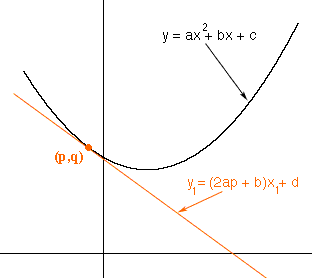Quandaries and Queries I have a question on the tangent to a quadratic curve. Say I have a curve y = ax2 + bx + c. The gradient, using the derivative of y, at any point x on the curve is: 2ax + b right? Then, for the tangent that cuts the curve at a point x, the equation of the tangent can be: y1 = (2ax + b)x1 + d. My question is, how is the point d of this tangent determined? It is the point on the y-axis where the tangent cuts isn't it? Is there a formula for it? Hi, I drew a diagram of what you described with one change in notation. Since you are using x as the independent variable to describe an arbitrary point on the parabola I used p as the first coordinate of the specific point on the parabola where you have constructed the tangent. I called the second coordinate of this point q.Since the point (p,q) is on the parabola you can find q, q = ap2 +bp + c The point (p,q) is also on the tangent line so the coordinates p and q satisfy the equation of the line. Thus q = (2ap + b)p + d and hence d = q - (2ap + b)p Penny Go to Math Central